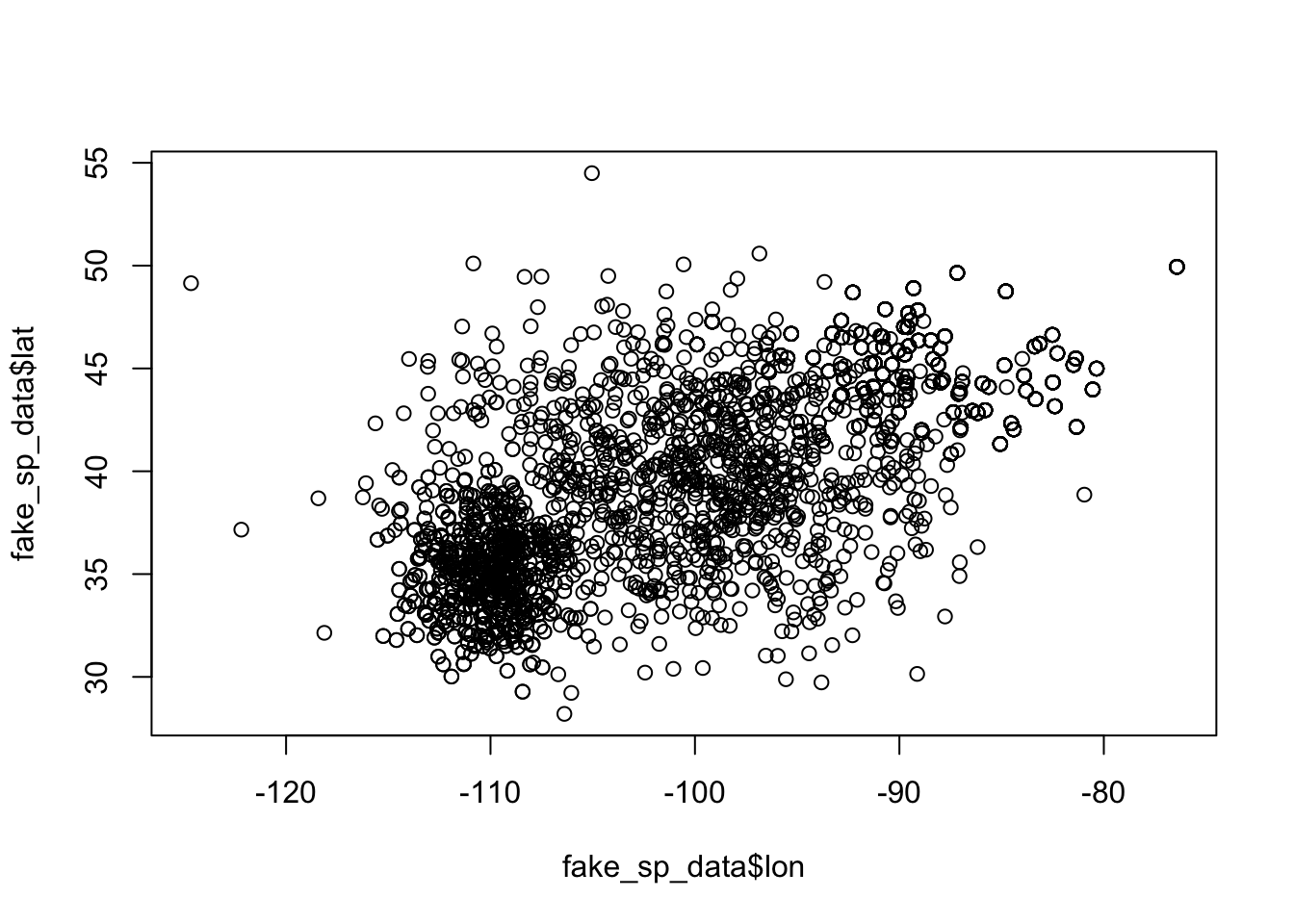# Mapping In R Part 1

Published:

Making maps with ggplot2 and dggridr

## Mapping in R

The purpose of this document is to help people get a jump start with mapping in R using dggridR and other helpful packages. If you have questions/comments, I would appreciate your input! btonelli (at) ucla [.] edu

First off, packages. You’ll need dplyr, devtools, and an older version of dggridR from the CRAN archive (the most recent version has some issues, or I don’t know how to use it correctly…). To do this, make sure you have devtools installed. You can skip this step once everything is installed.

library(devtools)
library(dplyr)
library(ggplot2)
#Uncomment and run the line immediately below if you haven't already!!
#install_version("dggridR", version = "2.0.4", repos = "http://cran.us.r-project.org")
library(dggridR)

## Making up some data

Next up, we will make some fake spatial data to work with. This will be data frame with 4 columns: lon, lat, species and beak length. We’ll simulate location and trait data for 3 fake species in North America. Each species will have a different spatial distribution and beak length.

wp_lat <- rnorm(1000,40,4)
warbler_lon <- rnorm(500,-110,2)
fake_sp_data <- rbind(data.frame(lon=rnorm(1000,-100,6),
lat=wp_lat,
species = rep("Drab Woodpecker",1000),
beak_length = rnorm(1000,(5+(wp_lat-40)/4),.5)),
data.frame(lon=warbler_lon,
lat=rnorm(500,35,2),
species = rep("Plain Warbler",1000),
beak_length = rnorm(500,3+(warbler_lon+110)/10,.25)),
data.frame(lon=rnorm(100,-90,5),
lat=rnorm(100,45,2),
species = rep("Fire-breasted Trainbearer",1000),
beak_length = rnorm(100,1.5,.1)))

fake_sp_data %>% group_by(species) %>% summarise(mean_beak_length = mean(beak_length))
## # A tibble: 3 × 2
##   species                   mean_beak_length
##   <chr>                                <dbl>
## 1 Drab Woodpecker                       4.96
## 2 Fire-breasted Trainbearer             1.50
## 3 Plain Warbler                         3.01

OK, so now lets do some basic plots to see where these birds are. In base R, we can see what it looks like if we just plot these birds out.

plot(fake_sp_data$lon,fake_sp_data$lat)Not super helpful! Lat and lon automatically scale, and it’s hard to get a sense of where these birds are across the continent. So next, let’s try to get the points plotted on a map. To do this, we will use ggplot2 and the function “map_data”

#First import country data.
#Because this data is located in the western hemisphere, we will filter to countries with longitudes less than -50 degrees
countries <- map_data("world") %>% filter(long < -30)

#Now plot using ggplot
basic_map1 <- ggplot() +
#This line adds the countries in, and colors the interior and border
geom_polygon(data=countries, aes(x=long, y=lat, group=group), fill="grey90", color="darkgrey")
plot(basic_map1)